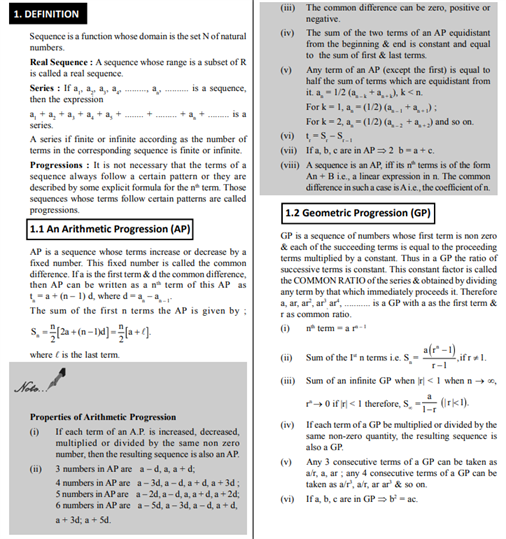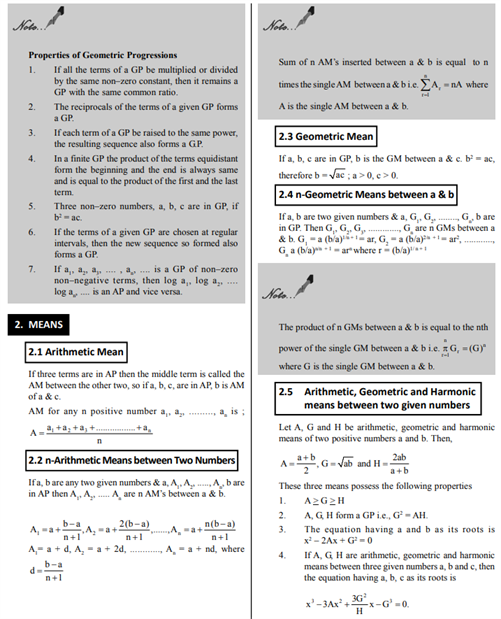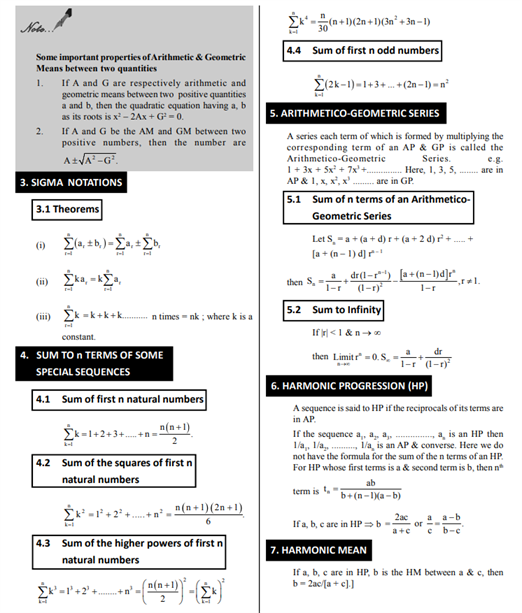# Revision Notes For CBSE Class 11 Maths Chapter 9 Sequence and Series

The class 11 Maths Revision Notes Chapter 9 Sequences and Series is one of the important chapters when it comes to understanding the basics of circular motion. We all are aware of the definition of sequence, Series and Progressions.

• The sequence is a function whose domain is the set N of natural numbers.
• Real Sequence: A sequence whose range is a subset of R is called a real sequence.
• Series : If a1, a2, a3, a4, ………, an, ………. is a sequence, then the expression a1 + a2 + a3 + a4 + a5 + …….. + ……… + an + ……… is a series.
• A series if finite or infinite according to as the number of terms in the corresponding sequence are finite or infinite.
• Progressions: It is not necessary that the terms of a sequence always follow a certain pattern or they are described by some explicit formula for the nth term. Those sequences whose terms follow certain patterns are called progressions.

In this notes, students will get to know the means, harmonic progressions, the arithmetico-geometric mean, harmonic mean. Preparing from this notes the important topics such as the theorem of the sigma notation will fetch a good score in exams.

Check out the maths revision notes for class 11 chapter 9 Sequences and Series here.